# Answered! MACM 316 Computing Assignment 2 1 of 2 Due Date: June 2nd, at 11pm. You must upload both your code (to…

#Matlab #GaussianElimination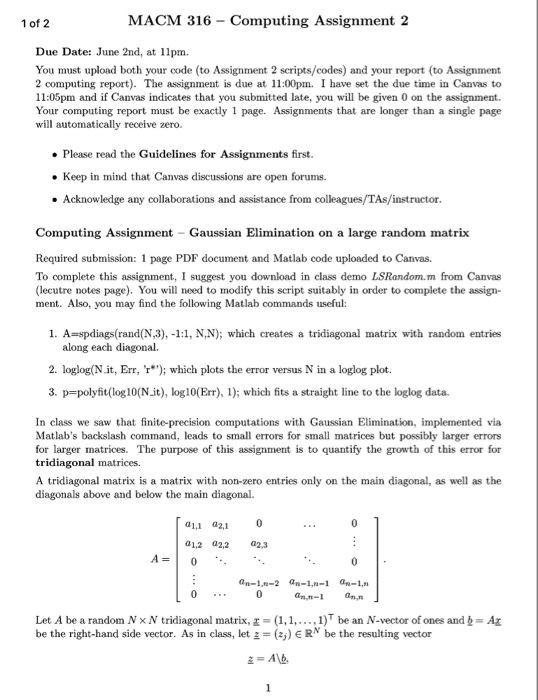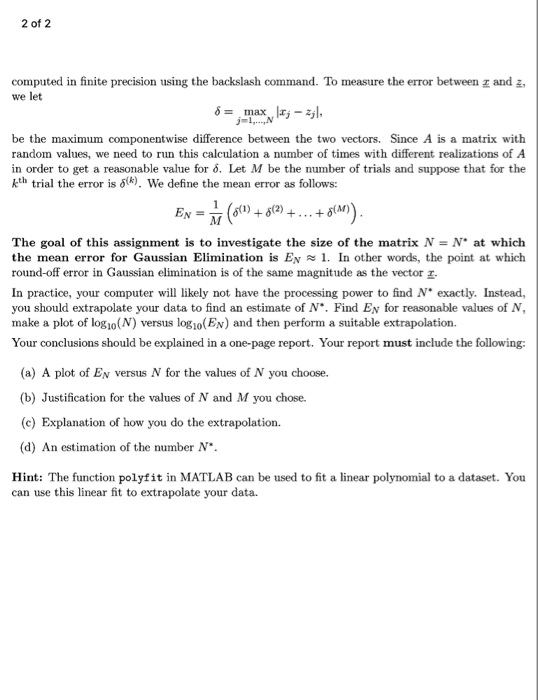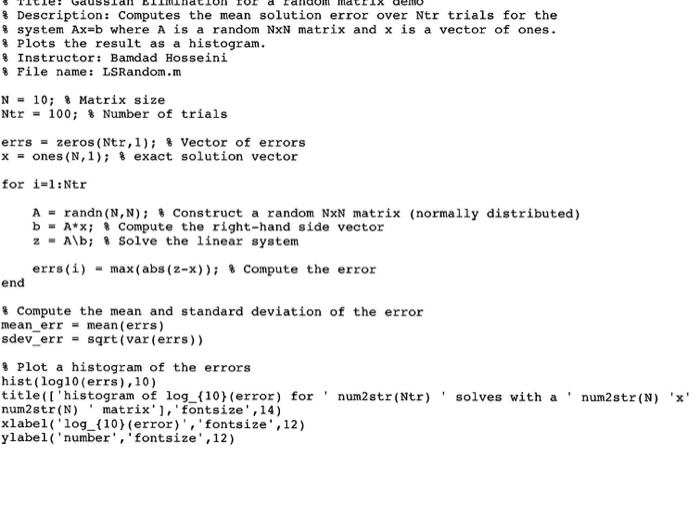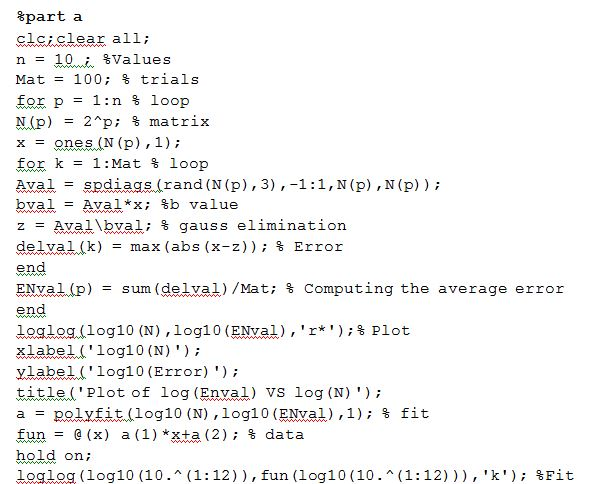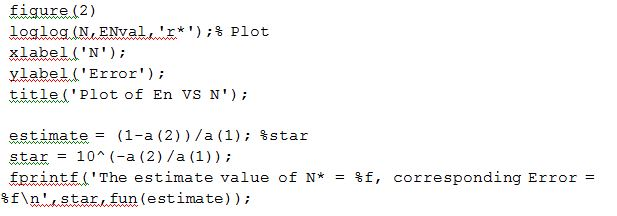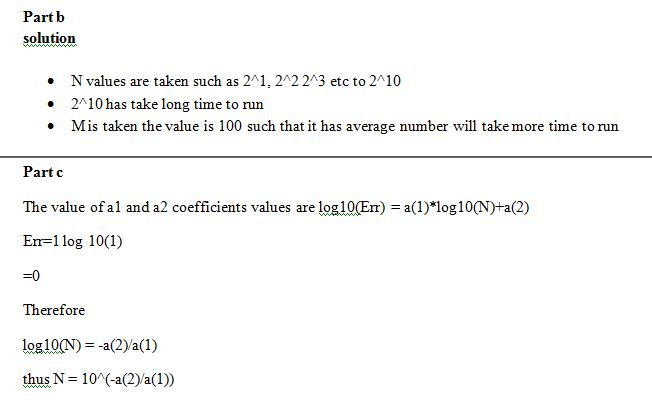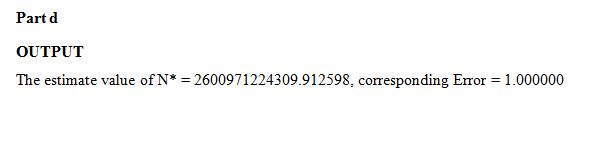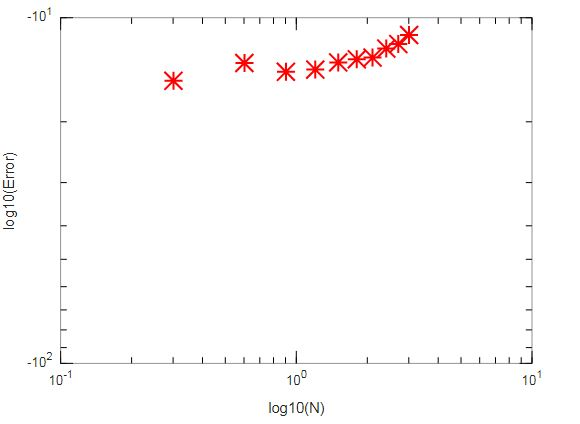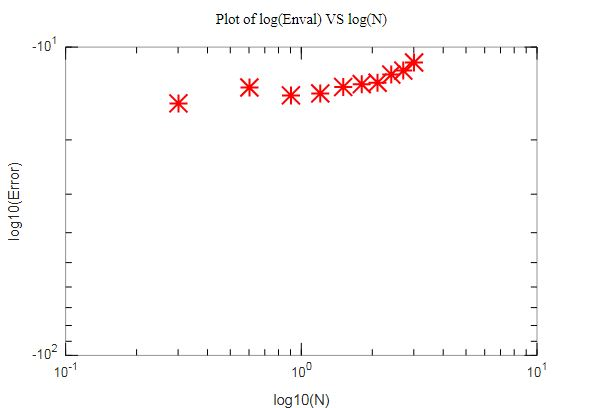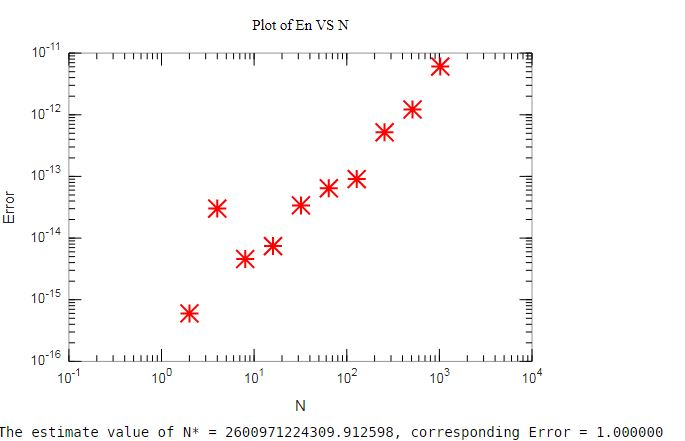Editable code:

%part a

Don't use plagiarized sources. Get Your Custom Essay on
Answered! MACM 316 Computing Assignment 2 1 of 2 Due Date: June 2nd, at 11pm. You must upload both your code (to…
GET AN ESSAY WRITTEN FOR YOU FROM AS LOW AS \$13/PAGE

clc;clear all;

n = 10 ; %Values

Mat = 100; % trials

for p = 1:n % loop

N(p) = 2^p; % matrix

x = ones(N(p),1);

for k = 1:Mat % loop

Aval = spdiags(rand(N(p),3),-1:1,N(p),N(p));

bval = Aval*x; %b value

z = Avalbval; % gauss elimination

delval(k) = max(abs(x-z)); % Error

end

ENval(p) = sum(delval)/Mat; % Computing the average error

end

loglog(log10(N),log10(ENval),’r*’);% Plot

xlabel(‘log10(N)’);

ylabel(‘log10(Error)’);

title(‘Plot of log(Enval) VS log(N)’);

a = polyfit(log10(N),log10(ENval),1); % fit

fun = @(x) a(1)*x+a(2); % data

hold on;

loglog(log10(10.^(1:12)),fun(log10(10.^(1:12))),’k’); %Fit

figure(2)

loglog(N,ENval,’r*’);% Plot

xlabel(‘N’);

ylabel(‘Error’);

title(‘Plot of En VS N’);

estimate = (1-a(2))/a(1); %star

star = 10^(-a(2)/a(1));

fprintf(‘The estimate value of N* = %f, corresponding Error = %fn’,star,fun(estimate));